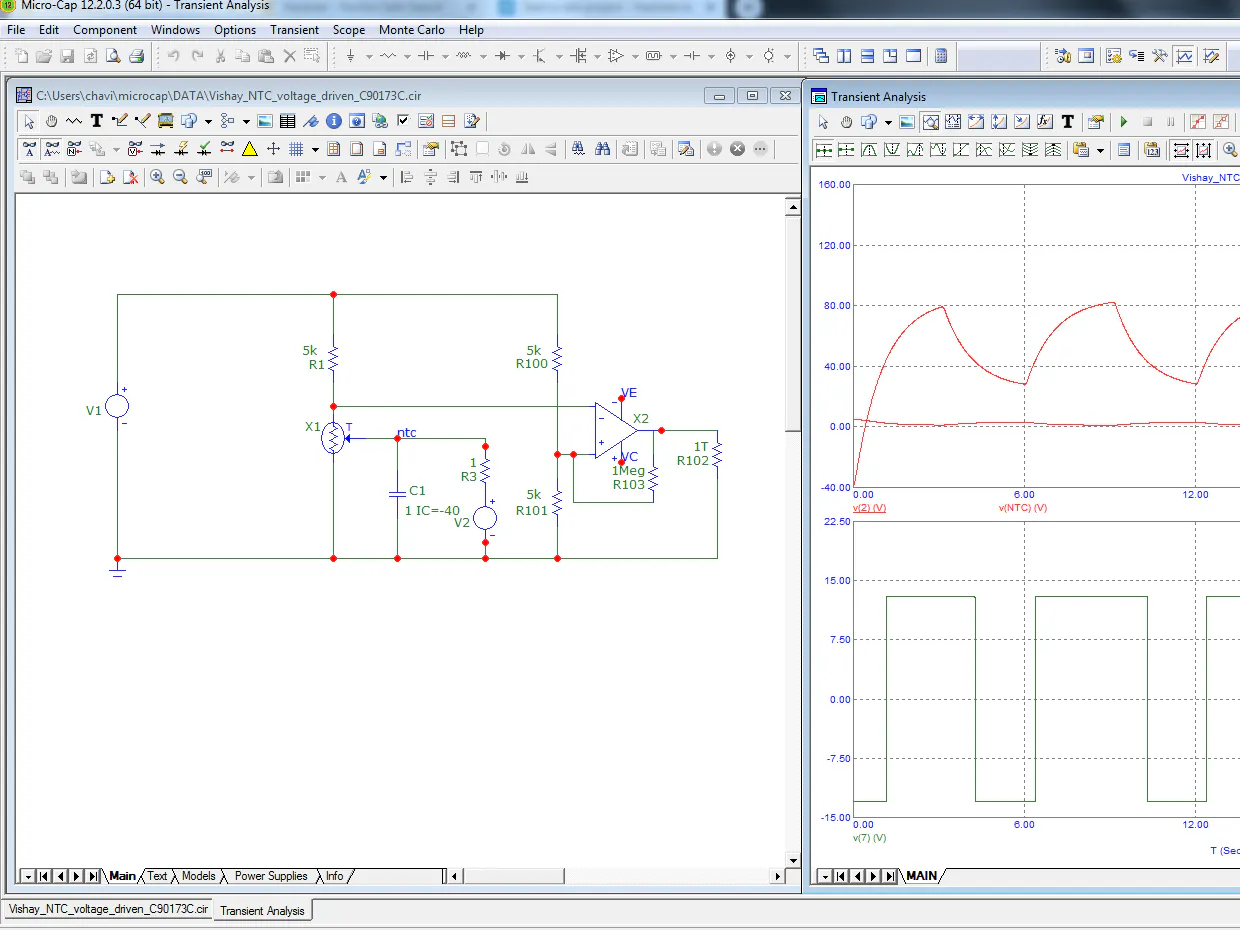# Dynamic modeling of a Vishay NTC thermistor in Micro-Cap

A circuit simulation is presented in the Micro-Cap software for a dynamic temperature measuring thermistor based Wheatstone bridge.

BeginnerProtip1 hour125## Things used in this project

### Hardware components

 Vishay NTCLE101E3C90173
×1
 Vishay TNPW06035K05BEEA
×1
 Texas Instruments uA741C
×1
 Vishay CRCW0603270KFKE
×1

## Schematics

### micro cap simulation of a thermistor based wheatstone bridge

open it in micro -cap

## Code

### netlist for a Vishay NTC themistor NTCLE101E3C90173 with temperature driven by voltage for micro-cap

Plain text
can be imported as text file as file.lib in microcap library
```.SUBCKT NTCLE101E3C90173 RN Rp Ti Params:
+ w=-13.40885683108
+ x=4547.96149408261
+ y=-176965.917057285
+ z=3861153.74690121
+ gth=0.0048 gth1 = 0.0000267
+ cth=0.08216
+ a=-13.408856831082
+ r25=10000
+ b=4547.96149408261
+ c=-176965.917057285
+ d=3861153.74690121
+ T0=273.15
G_G1         AOUT 0 VALUE { if(V(Ti)>25,V(AOUT,
+  0)/(R25*exp(((D/(T0+abs(V(H))+V(Ti))+C)/(T0+abs(V(H))+V(Ti))+B)/(T0+V(Ti)+abs(V(H)))+A)),0)
+  }
G_G2         AOUT 0 VALUE { IF(V(Ti)>25,0,V(AOUT,
+  0)/(R25*exp(((Z/(T0+abs(V(H))+V(Ti))+Y)/(T0+abs(V(H))+V(Ti))+X)/(T0+abs(V(H))+V(Ti))+W)))
+  }
G_G3         H 0 VALUE {
+  if(V(Ti)>25,-V(RP,RN)*V(RP,RN)/(R25*exp(((D/(T0+abs(V(H))+V(Ti))+C)/(T0+abs(V(H))+V(Ti))+B)/
+  (T0+V(Ti)+abs(V(H)))+A)),0)}
G_G4         H 0 VALUE {
+  if(V(Ti)>25,0,-V(RP,RN)*V(RP,RN)/(R25*exp(((Z/(T0+abs(V(H))+V(Ti))+Y)/(T0+abs(V(H))+V(Ti))+X)/
+  (T0+V(Ti)+abs(V(H)))+W)))}
G_G5         RP RN VALUE { V(RP, RN)/V(AOUT) }
G_G6         H 0 VALUE { V(H)*(Gth + Gth1*(V(Ti)-25)) }
R_R1         0 AOUT  1T TC=0,0
R_R2         0 H  1T TC=0,0
C_C1         0 H  {Cth}
R_R99        0 Ti 1T
.IC V(H) =0
.ENDS
```

## Credits

### alainstas

13 projects • 4 followers
product engineer at Vishay